# Discount Factor

Discount Factor Formula and Calculation Steps Explained (Excel Template Included)Key Learning Objectives
• What is the formula for calculating the discount factor?
• What is the purpose of the discount factor?
• What is the relationship between the discount factor and the present value?

## Discount Factor Formula: Approach 1

Generally speaking, there are two approaches to calculating the discount factor, but in either case, the discount factor is a function of the:

1. Discount Rate
2. Time Period

The discount rate can be thought of as representing the percentage of return that you could have received by investing that dollar, if you had received it today.

The reason you would prefer to have \$1 today than \$1 three years from now is because if you received the \$1 three years from now, you would have missed out on a full three years where you could have invested that \$1 and ended up with more than \$1 by the end of that time.

The first formula for the discount factor has been shown below.And the formula can be re-arranged as:Either formula could be used in Excel; however, we will be using the first formula in our example as it is a bit more convenient (i.e., Excel re-arranges the formula itself in the first formula).

To arrive at the present value using the first approach, the factor would then be multiplied by the cash flow to get the present value (“PV”).While the discount rate remains constant throughout the projection, the period number rising is what causes the factor to decrease over time.

Note that the period can be whatever length you want (years, months, days, even hours) – but it is critical to ensure that the period is aligned with the implied period of the discount rate.

Intuitively, the discount factor, which is always calculated by one divided by a figure, decreases the cash flow values. This also ties back to what we discussed in the beginning, where receiving \$1 today is more valuable than receiving \$1 in the future.

To tie this back to the example using \$1, assuming a 10% discount rate and a one-year time horizon – the discount factor would be calculated as:

• 0.91 = 1 / (1 + 10%) ^ 1

Next, the present value can be calculated using:

• 0.91 = \$1 × 0.91

The example implies that \$1 dollar received one year from the current period would be worth \$0.91 in the present day.

## Discount Factor Formula: Approach 2

The formula for the second approach is virtually identical except for the absence of the negative sign in front of the period number exponent.Unlike the first approach, the present value formula this time around divides the cash flow by the discount factor.By entering the discount factor formula into the PV formula, the formula can be re-expressed as:As opposed to decreasing over time, the factor increases in this case – thereby, the downward adjustment on the present value becomes more apparent in later years.

Returning back to the \$1 dollar example with the same 10% discount rate and one-year time frame, the calculation is:

• 1.1 = (1 + 10%) ^ 1

And upon applying this to the \$1 in cash flow:

• 0.91 = 1 / 1.1

So, as we can see, both methods calculate the same present value for the \$1 one year from today (\$0.91).

Now that we have explained the formulas, we are now ready to implement them into Excel.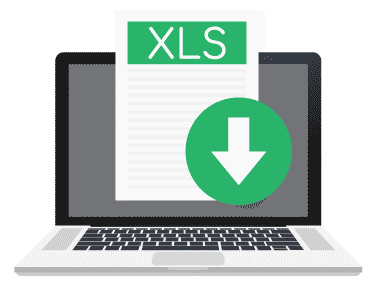Submitting ...

## Discount Factor Calculation: Approach 1

In the hypothetical scenario we will be using, the company has the following financial profile:

• Cash Flow: \$100/Year
• Discount Rate: 10%

For example, in 2021, the discount factor comes out to 0.91 after adding the 10% discount rate to 1 and then raising the amount to the exponent of -1, which is the matching time period.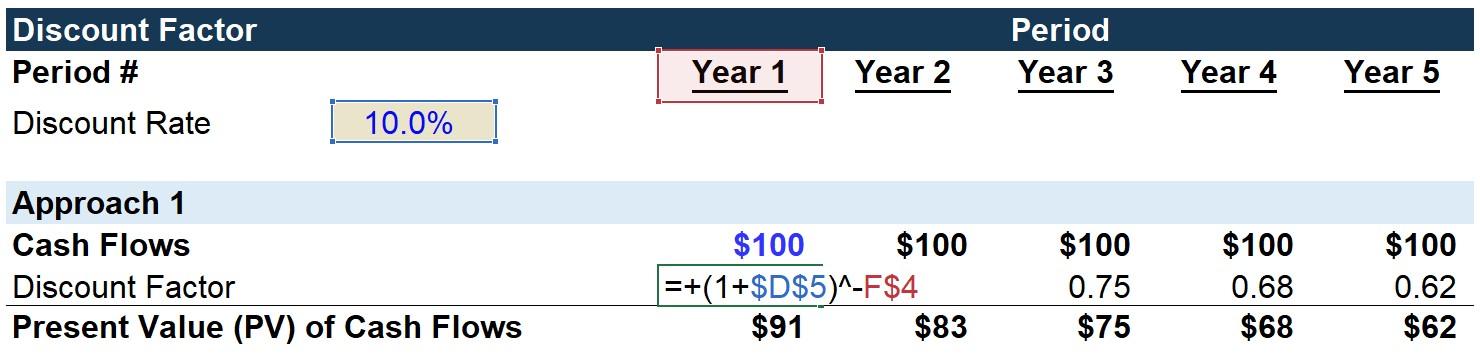The 0.91 is subsequently multiplied by the cash flow of \$100 to get \$91 as the PV of the 1st year cash flow.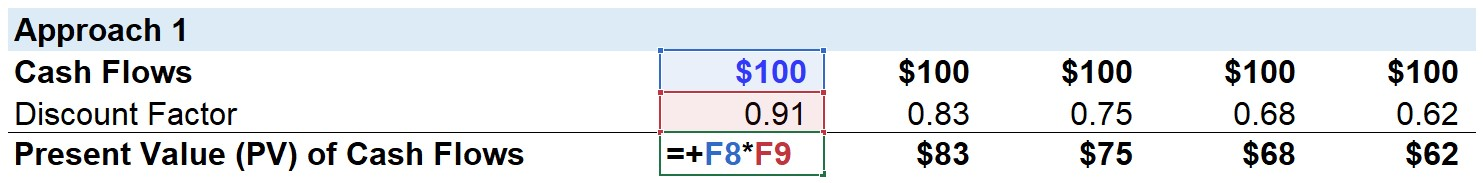By the end of Year 5, we can see the discount factor drops in value from 0.91 to 0.62 by the end of the forecast period due to the time value of money.

## Discount Factor Calculation: Approach 2

Recall how this time around, the cash flow will be divided by the discount factor to get the present value.

And in contrast to the 1st approach, the factor will be in excess of 1.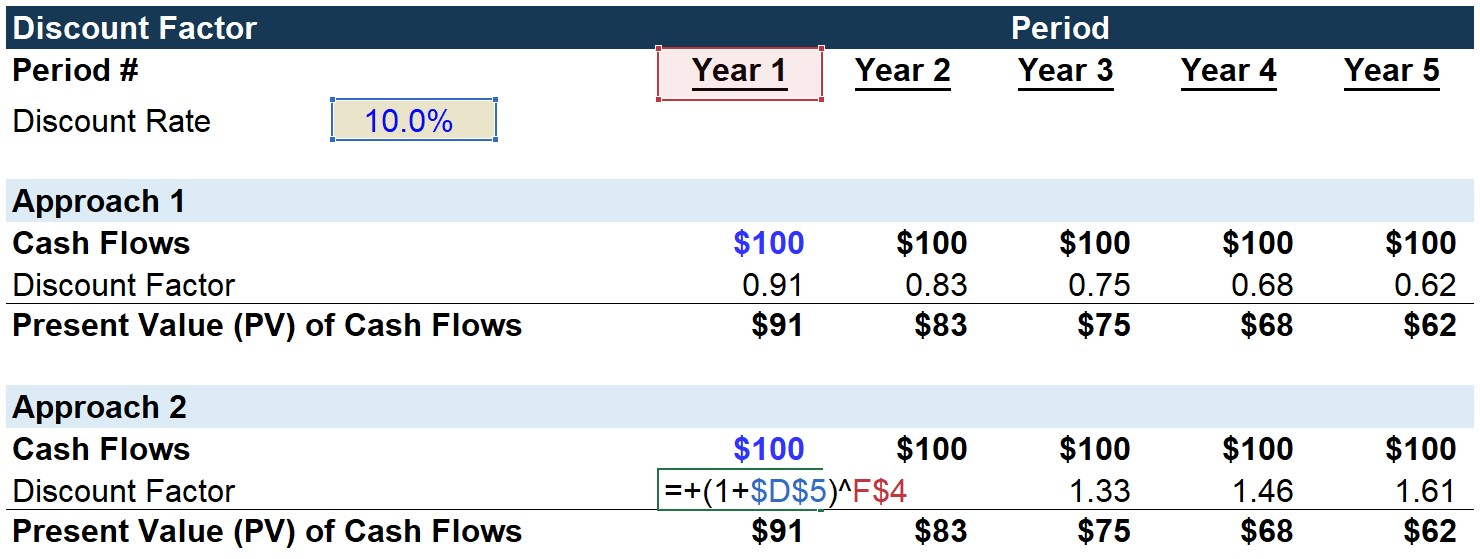For 2021, the discount rate of 10% is added to 1, which is raised to the exponent of 1, as that is the first projected year. From doing so, the output is 1.10.

Conversely, the factor increases over time in the 2nd approach since the cash flows are being divided by this >1 factor.

Then, the 1st year cash flow of \$100 is divided by 1.10 to get \$91 for the PV of the cash flow.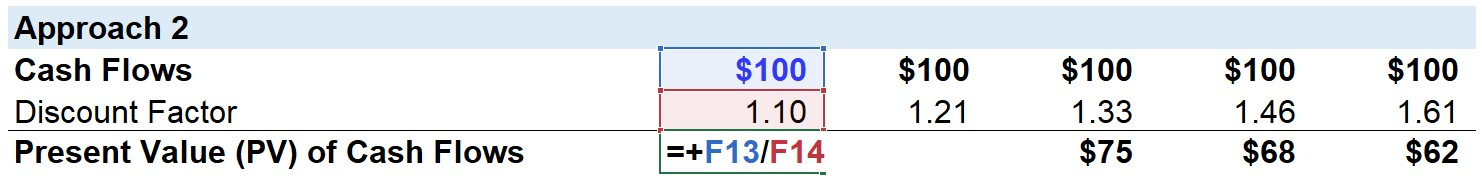Here, in the finished output sheet below, the present values of the cash flows calculated under both approaches result in the same figures.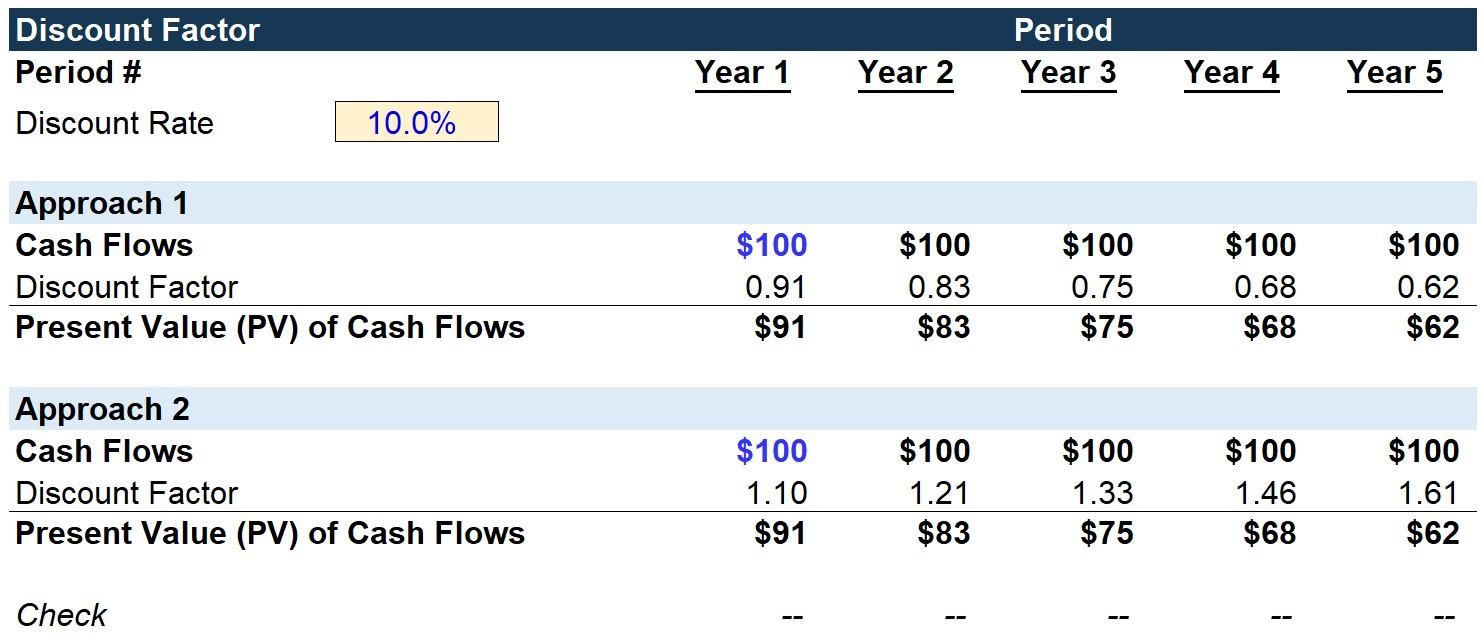Ultimately, it does not matter which approach you decide to take, because conceptually the rationale and impact of the discount factor are exactly identical.Inline FeedbacksLearn Financial Modeling Online

Learn to build a 3-statement model using various supporting schedules and best practices.

X

The Wall Street Prep Quicklesson Series

7 Free Financial Modeling Lessons

Get instant access to video lessons taught by experienced investment bankers. Learn financial statement modeling, DCF, M&A, LBO, Comps and Excel shortcuts.